## In a pickup game of dorm shuffleboard, students crazed by final exams use a broom to propel a calculus book along the dorm hallway. If the 3

Question

In a pickup game of dorm shuffleboard, students crazed by final exams use a broom to propel a calculus book along the dorm hallway. If the 3.5 kg book is pushed from rest through a distance of 0.90 m by the horizontal 25 N force from the broom and then has a speed of 1.60 m/s, what is the coefficient of kinetic friction between the book and floor?

in progress 0
5 months 2021-08-27T21:08:50+00:00 2 Answers 43 views 0

## Answers ( )

μk = 0.58

Explanation:

• As the book starts from rest, till it reaches to a given speed, this means thar -according Newton’s 2nd law- there must be a net force pushing it.
• If the broom exerts a constant horizontal force of 25N, we can find if this is a only force acting on the book, finding first the acceleration of the book.
• As it starts from rest,  the initial speed is v₀=0. We know the value of the final speed vf, and the distance travelled Δx, we can use the following kinematic equation in order to find the acceleration a: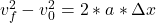• Replacing by the givens, we can find a as follows: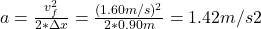• Applying Newton’s 2nd law, we can find the total net force on the book, as follows: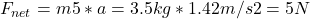• So, if the applied force is 25 N, and the net force is only 5N, this means that there must be another force, opposing to the applied force, of 20 N.
• This force is just the kinetic friction force, opposing to the relative movement between the surfaces in contact (the book and the dorm hallway floor), and is given by the following expression: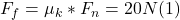• where μk = coefficient of kinetic friction between the book and the floor (our unknown), and Fₙ, is the normal force.
• The normal force, can adopt any value needed to prevent a vertical net acceleration, and is always perpendicular to the surface of contact, and upward from the surface.
• In this case, this normal force is just equal and opposite to the force exerted by gravity: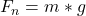• Replacing this value by the givens in (1) , we can solve for μk, as follows: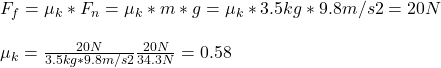• The coefficient of kinetic friction between the book and the floor is 0.58.

μ = 0.145

Explanation:

For this this problem let’s start by using the kinematic relations to find the acceleration of the body

v² = v₀² + 2 a x

As part of rest v₀ = 0

a = v² / 2x

a = 1.60²/2 0.90

a = 1.42 m / s²

Now we can use Newton’s second law

fr = m a

N- W = 0

The equation for friction force is

fr = μ N

fr = μ m g

We replace

μ m g = m a

μ = a / g

μ = 1.42 /9.80

μ = 0.145